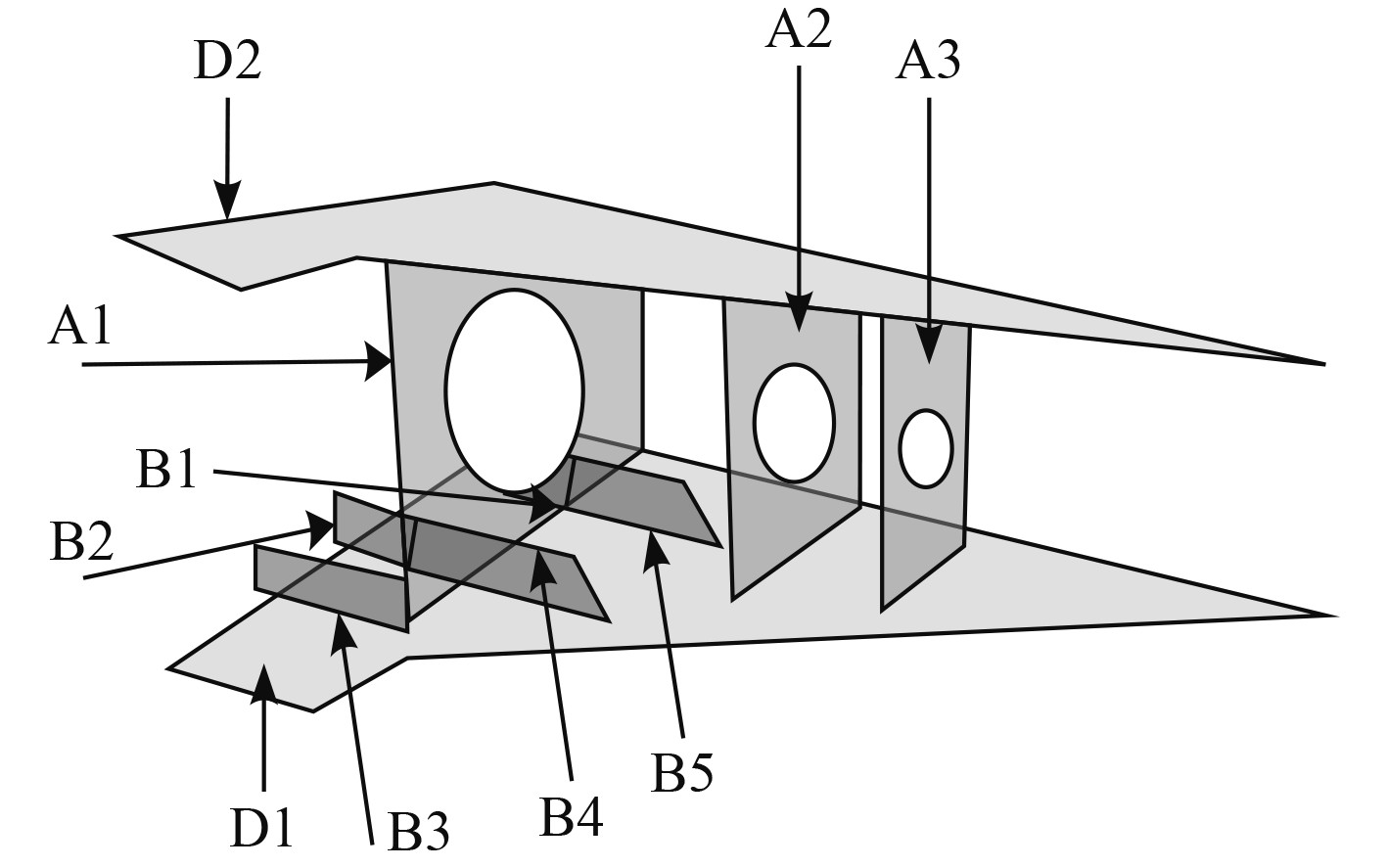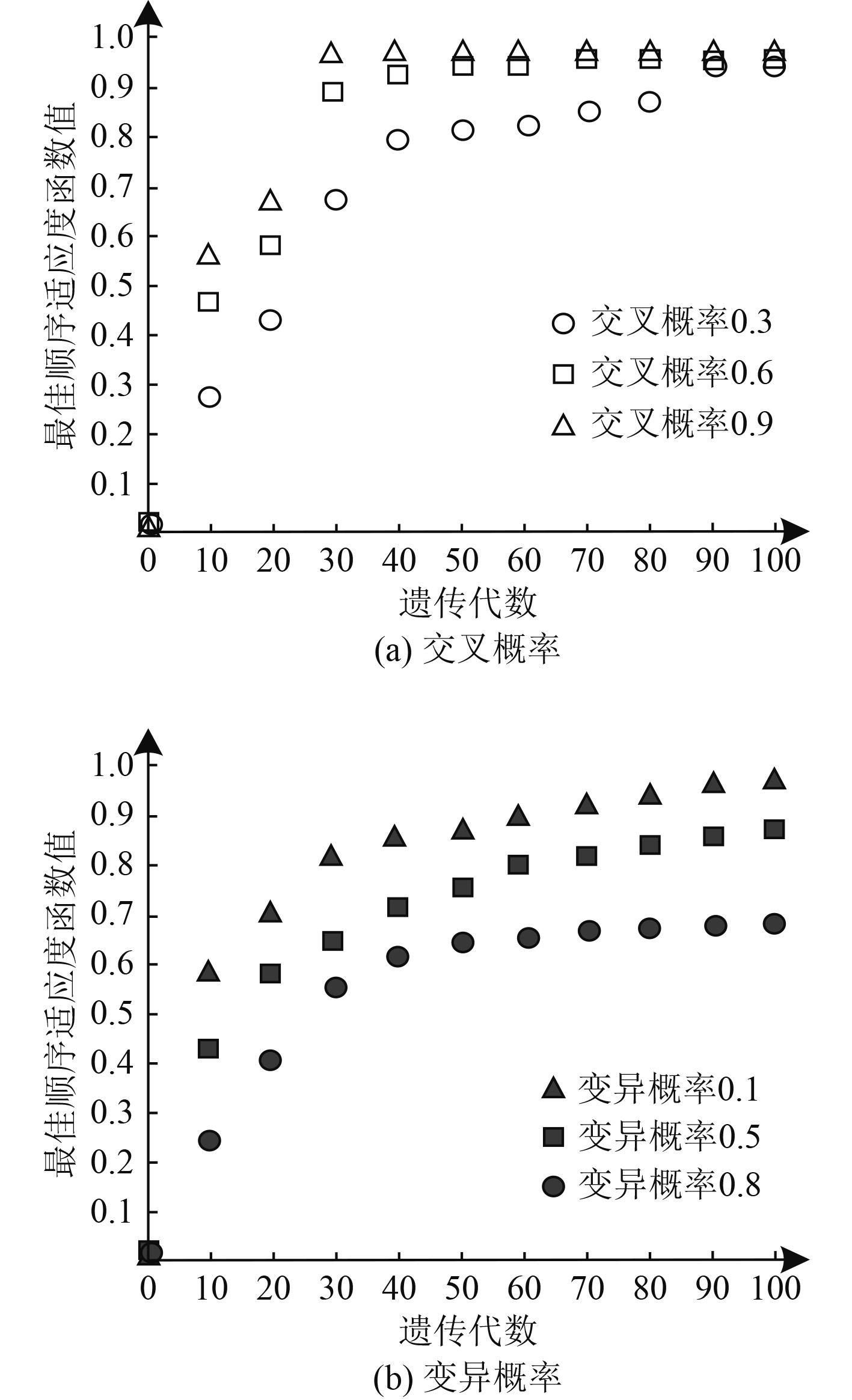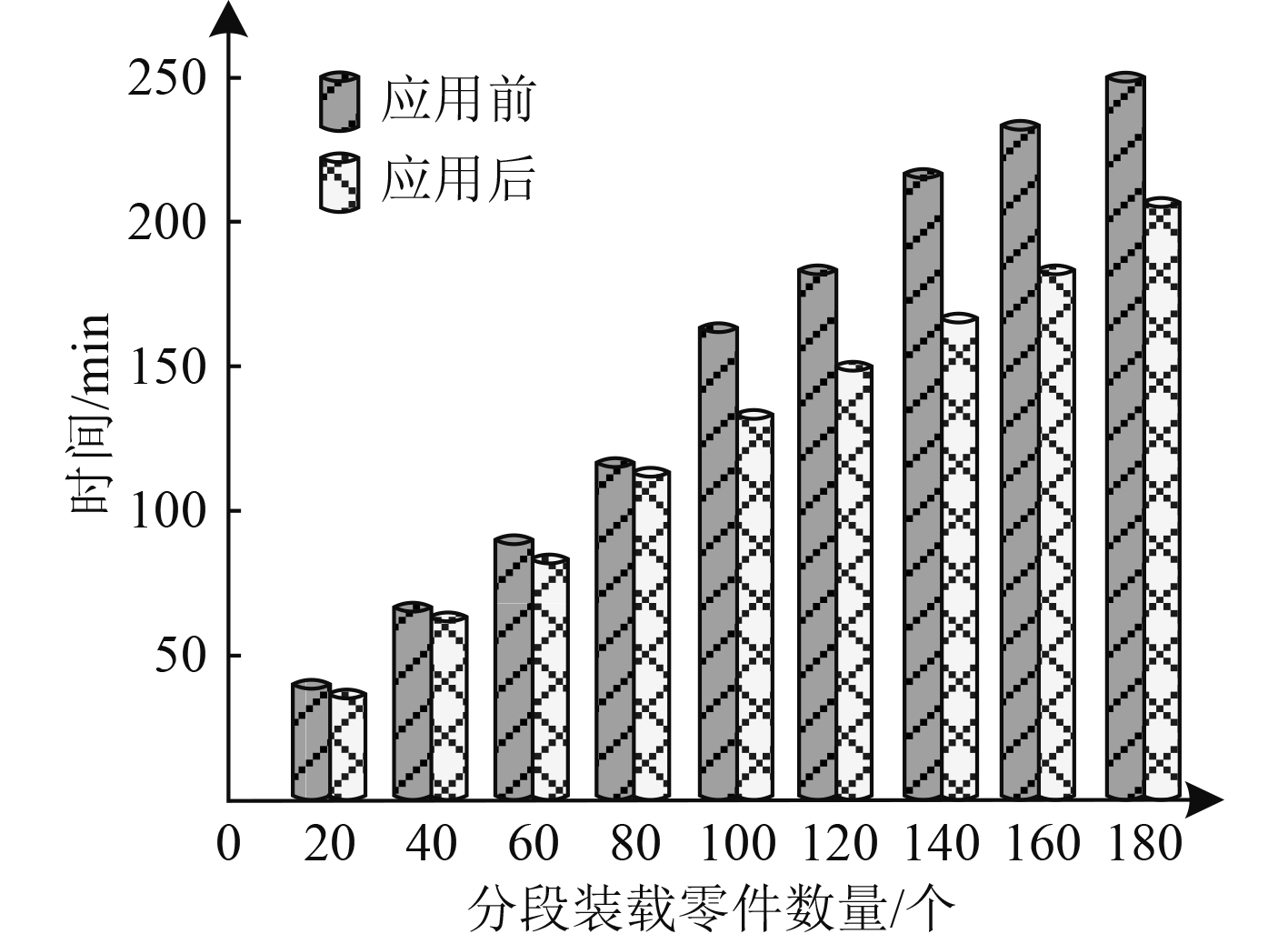﻿ 基于遗传模拟退火算法的舰船分段装载顺序优化设计
 舰船科学技术2022, Vol. 44Issue (5): 150-153    DOI: 10.3404/j.issn.1672-7649.2022.05.032PDF

Sequence optimization design of ship sectional loading based on genetic simulated annealing algorithm
ZHANG Xiao-ling
Henan Wisdom Education and Intelligent Technology Application Engineering Technology Research Center, Zhengzhou 451460, China
Abstract: In order to reduce the frequency of interference and tool change, a sequence optimization method based on genetic simulated annealing algorithm was designed. Piecewise load sequence optimization mathematical model is built with, and set up the contact relation between the parts and components assembly sequence feasibility of intervention, by using the genetic simulated annealing algorithm by selecting fitness function, selection section load factor, crossover and mutation operation, and simulated annealing processing steps, such as solving block loading sequence optimization mathematical model of the objective function. The optimization results of ship loading sequence are obtained. Experimental results show that the optimal crossover probability and variation probability are 0.9 and 0.1, respectively. After the optimization, the cumulative interference times and the cumulative tool replacement times of ship loading sequence are reduced three times and four times, respectively. The optimization effect is significant.
Key words: genetic simulated annealing     ship sectional loading     sequential optimization design     mathematical model     feasibility conditions
0 引　言

1 舰船分段装载的顺序优化方法 1.1 分段装载顺序优化数学模型建立

 $\mathrm{min}S=({\sigma }_{1}+{\sigma }_{2}+{\sigma }_{3}+{\sigma }_{4})\cdot({f}_{1}+{f}_{2}+{f}_{3}+{f}_{4}) 。$ (1)

1.2 装配顺序可行性条件设置

 $A{S_m} = \left\{ {{Q_1},{Q_2}, \cdots ,{Q_m}} \right\} 。$ (2)

1）装载顺序零件接触关系可行性设置

$i$ 个分段零件装载之初，先考虑与该分段零件其他存在关联关系分段零件是否已经装载，若是，则第 $i$ 个分段零件在该装载顺序较为合理。反之则认为第 $i$ 个分段零件在其装载顺序内不可行。舰船在分段装载时，其第一个分段零件无须考虑上述接触关系，但其后续的每个零件均需考虑与之存在关联关系的是否已经装载。在判断舰船分段零件之间的关联关系时，利用矩阵形式描述，其表达式如下：

 ${M_A} = {\left[ {{M_{ij}}} \right]_{N\cdot N}} 。$ (3)

 ${M_i} = {M_i}{Q_1} \cup {M_i}{Q_2} \cup {M_i}{Q_3} \cup \cdots \cup {M_i}{Q_m}。$ (4)

2）装载顺序干涉可行性设置

 $Y = \left\{ {{b_{ijX}},{b_{ijY}},{b_{ijZ}},{b_{jiX}},{b_{jiY}},{b_{jiZ}}} \right\} 。$ (5)

 ${b_{dk}} = {\left[ {{b_{ij{d_k}}}} \right]_{N*N}} 。$ (6)

 $\left\{ \begin{gathered} + {b_{i{d_k}}} = {b_{i{Q_1}{d_k}}} \cup {b_{i{Q_2}{d_k}}} \cup \cdots {b_{i{Q_m}{d_k}}}，\hfill \\ - {b_{i{d_k}}} = {b_{{Q_1}i{d_k}}} \cup {b_{{Q_2}i{d_k}}} \cup \cdots {b_{{Q_m}i{d_k}}} 。\hfill \\ \end{gathered} \right.$ (7)

1.3 基于遗传模拟退火算法的分段装载顺序优化数学模型求解

1）适应度函数选取

 $AU = \sum\limits_{i = 1}^n {({U_{{x_{i - 1}},{x_i}}})}，$ (8)
 ${U_{{x_{i - 1}},{x_i}}} = {\alpha _i} + {\beta _i} + {\gamma _i} + {\omega _i} 。$ (9)

 $Fitness({Q_i}) = \sum\limits_{i = 1}^N {4i - \sum\limits_{i = 1}^N {({\alpha _i} + {\beta _i} + {\gamma _i} + {\omega _i})} }。$ (10)

2）分段装载因子选择

 ${E_i} = \frac{{{h_i}}}{{\displaystyle\sum\limits_{j = 1}^N {{h_j}} }}。$ (11)

 $\sum\limits_{j = 0}^{i - 1} {{E_j}} \leqslant r \leqslant \sum\limits_{j = 0}^i {{E_j}} 。$ (12)

3）交叉与变异操作

4）模拟退火处理

 ${E_a} = \left\{ \begin{gathered} 1,{F_i}(O) < {F_i}(O)，\hfill \\ \exp \left[ {\frac{{{F_i}(O)}}{T} - \frac{{{F_j}(O)}}{T}} \right],{F_i}(O) \geqslant {F_i}(O)。\hfill \\ \end{gathered} \right.$ (13)

 $O = O\cdot \xi，$ (14)

5）建立初始因子群并设置迭代终止条件。利用优先级函数和随机数函数各生成一半初始因子群，其中将随机数生成的因子作为优先级，其计算公式如下：

 ${E_i} =\left (\frac{{{l_i}{p_r}}}{{{w_i}}} + \frac{{{l_i}\cdot {w_i}\cdot {E_a}}}{{{w_i}}}\right)。$ (16)

2 实验结果分析图 1 自升式起重船船底边仓组件结构示意图 Fig. 1 structural diagram of bottom side bin components of jack up crane ship
2.1 交叉与变异概率确定图 2 交叉概率和变异概率确定结果 Fig. 2 Determination results of crossover probability and mutation probability
2.2 分段装载的顺序优化测试图 3 不同分段装载零件数量时优化前后所用时间 Fig. 3 time before and after optimization when loading parts in different sections
3 结　语

  朱杰, 张文怡, 薛菲. 基于遗传模拟退火算法的立体仓库储位优化[J]. 计算机应用, 2020, 40(1): 284-291.  王国武, 陈元琰. 基于跳数修正和遗传模拟退火优化DV-Hop定位算法[J]. 计算机科学, 2021, 48(S1): 313-316.  袁福帅, 余震, 朱浩涛, 等. 基于改进遗传算法的航材装载优化[J]. 包装工程, 2021, 42(23): 249-258.  李国杰, 丁晓红. 智能起重机货物装卸顺序优化与自动生成技术[J]. 机械工程学报, 2020, 56(18): 254-264.  CHU Ding-li, CHENG Hong, WANG Xu-guang. Whale optimization algorithm based on adaptive weight and simulated annealing[J]. Acta Electronica Sinica, 2019, 47(5): 992-999.  陈卓, 冯钢, 刘怡静, 等. MEC中基于改进遗传模拟退火算法的虚拟网络功能部署策略[J]. 通信学报, 2020, 41(4): 70-80. DOI:10.11959/j.issn.1000-436x.2020074  张长勇, 张倩倩, 翟一鸣, 等. 基于改进粒子群算法的航空行李在线装载优化[J]. 包装工程, 2021, 42(21): 200-206.  吴伟, 邓准, 尚建忠, 等. 精密光机系统多敏感轴装配精度分析与装配工艺优化[J]. 农业机械学报, 2021, 52(4): 418-426. DOI:10.6041/j.issn.1000-1298.2021.04.046  杨艳芳, 杨秒, 舒亮, 等. 考虑工序刚性约束的自动化装配生产线多目标优化研究[J]. 机械工程学报, 2020, 56(7): 181-192.  宣传伟, 韩景龙. 非结构重叠网格显式装配算法[J]. 北京航空航天大学学报, 2019, 45(10): 2026-2034.  周鹏, 董朝轶, 陈晓艳, 等. 基于阶梯式Tent混沌和模拟退火的樽海鞘群算法[J]. 电子学报, 2021, 49(9): 12-17.